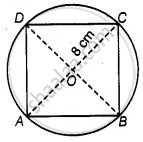# The area of the square that can be inscribed in a circle of radius 8 cm is ______. - Mathematics

MCQ
Fill in the Blanks

The area of the square that can be inscribed in a circle of radius 8 cm is ______.

#### Options

• 256 cm2

• 128 cm2

• 64sqrt(2) cm2

• 64 cm2

#### Solution

The area of the square that can be inscribed in a circle of radius 8 cm is 128 cm2.

Explanation:

Given, radius of circle, r = OC = 8cm.

∴ Diameter of the circle = AC = 2 × OC = 2 × 8 = 16 cm

Which is equal to the diagonal of a square.

Let side of square be x.In right-angled ΔABC,

AC2 = AB2 + BC2   ......[By Pythagoras theorem]

⇒ (16)^2 = x^2 + x^2

⇒ 256 = x^2 + x^2

⇒ x^2 = 128

∴ Area of square = x^2 = 128  cm^2

Alternative Method:

Radius of circle (r) = 8 cm

Diameter of circle (d) = 2r = 2 × 8 = 16 cm

Since, square inscribed in circle.

∴ Diagonal of the square = Diameter of circle

Now, Area of square = ("Diagonals")^2/2

= (16)^2/2

= 256/2

= 128 cm2

Concept: Area of Circle
Is there an error in this question or solution?

#### APPEARS IN

NCERT Mathematics Exemplar Class 10
Chapter 11 Area Related To Circles
Exercise 11.1 | Q 8 | Page 121

Share